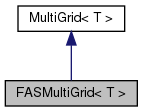Chombo + EB  3.2
FASMultiGrid< T > Class Template Reference

`#include <FASMultiGrid.H>`

Inheritance diagram for FASMultiGrid< T >:[legend]

## Public Member Functions

FASMultiGrid ()

virtual ~FASMultiGrid ()

virtual void define (MGLevelOpFactory< T > &a_factory, LinearSolver< T > *a_bottomSolver, const ProblemDomain &a_domain, int a_maxDepth=-1, MGLevelOp< T > *a_finestLevelOp=NULL)
Function to define a FASMultiGrid object. More...

virtual void solve (T &a_phi, const T &a_rhs, Real a_tolerance, int a_maxIterations, int verbosity=0)

virtual void oneCycle (T &a_e, const T &a_res)
Execute ONE v-cycle of multigrid. More...

void oneCycle (T &a_e, const T &a_res, T *a_phiCoarse)

virtual void cycle (int a_depth, T &a_correction, const T &a_residual)Public Member Functions inherited from MultiGrid< T >
MultiGrid ()

virtual ~MultiGrid ()

void init (const T &a_correction, const T &a_residual)

void cycle (int a_depth, T &a_correction, const T &a_residual)

void clear ()

void setBottomSolver (LinearSolver< T > *a_bottomSolver)

Vector< MGLevelOp< T > *> getAllOperators ()

## Protected Attributes

Vector< T *> m_phiCoarseProtected Attributes inherited from MultiGrid< T >
bool m_defined

int m_bottomCells

Vector< MGLevelOp< T > * > m_op

Vector< T *> m_residual

Vector< T *> m_correction

std::vector< bool > m_ownOpPublic Attributes inherited from MultiGrid< T >
int m_depth

int m_defaultDepth

int m_pre

int m_post

int m_bottom

int m_cycle

int m_numMG

bool m_homogeneous

LinearSolver< T > * m_bottomSolver

ProblemDomain m_topLevelDomain

## ◆ FASMultiGrid()

template<class T >
 FASMultiGrid< T >::FASMultiGrid ( )

## ◆ ~FASMultiGrid()

template<class T >
 FASMultiGrid< T >::~FASMultiGrid ( )
virtual

References CH_TIME.

## ◆ define()

template<class T >
 void FASMultiGrid< T >::define ( MGLevelOpFactory< T > & a_factory, LinearSolver< T > * a_bottomSolver, const ProblemDomain & a_domain, int a_maxDepth = `-1`, MGLevelOp< T > * a_finestLevelOp = `NULL` )
virtual

Function to define a FASMultiGrid object.

a_factory is the factory for generating operators. a_bottomSolver is called at the bottom of v-cycle. a_domain is the problem domain at the top of the vcycle. maxDepth defines the location of the bottom of the v-cycle. The vycle will terminate (hit bottom) when the factory returns NULL for a paticular depth if maxdepth = -1. Otherwise the vycle terminates at maxdepth.

Reimplemented from MultiGrid< T >.

References MultiGrid< T >::define(), and MultiGrid< T >::m_homogeneous.

## ◆ solve()

template<class T >
 void FASMultiGrid< T >::solve ( T & a_phi, const T & a_rhs, Real a_tolerance, int a_maxIterations, int verbosity = `0` )
virtual

solve L(a_phi) = a_rhs. Tolerance is how much you want the norm of the error reduced. verbosity is how chatty you want the function to be. maxIterations is the maximum number of v-cycles. This does the whole residual correction switcharoo and calls oneCycle up to maxIterations times, evaluating the residual as it goes.

Reimplemented from MultiGrid< T >.

## ◆ oneCycle() [1/2]

template<class T >
 void FASMultiGrid< T >::oneCycle ( T & a_e, const T & a_res )
virtual

Execute ONE v-cycle of multigrid.

If you want the solution to converge, you need to iterate this. See solve() or AMRFASMultiGrid::solve for a more automatic solve() function.

Reimplemented from MultiGrid< T >.

References CH_assert.

Referenced by AMRFASMultiGrid< T >::VCycle().

## ◆ oneCycle() [2/2]

template<class T >
 void FASMultiGrid< T >::oneCycle ( T & a_e, const T & a_res, T * a_phiCoarse )

## ◆ cycle()

template<class T >
 void FASMultiGrid< T >::cycle ( int a_depth, T & a_correction, const T & a_residual )
virtual

## ◆ m_phiCoarse

template<class T>
 Vector< T* > FASMultiGrid< T >::m_phiCoarse
protected

The documentation for this class was generated from the following file: